﻿ General Formulation of Ångström-Prescott Coefficients: A New Approach for Côte d’IvoirePublications are Open
Access in this journal
Article Versions
Export Article
• Normal Style
• MLA Style
• APA Style
• Chicago Style
Research Article
Open Access Peer-reviewed

### General Formulation of Ångström-Prescott Coefficients: A New Approach for Côte d’Ivoire

Maurice Aka Djoman , Ferdinand Wanignon Fassinou, Augustin Memelèdje
International Journal of Physics. 2021, 9(5), 245-250. DOI: 10.12691/ijp-9-5-4
Received July 22, 2021; Revised August 23, 2021; Accepted September 02, 2021

### Abstract

In this study, we used calibrated annual Ångström-Prescott (AP) coefficients of nine (9) weather stations in Côte d'Ivoire to formalize two general equations expressing them as a function of the latitude and altitude. The aim is formalizing the general equations of Ångström-Prescott coefficients that can be used to increase the density of global horizontal irradiance. The Ångström-Prescott coefficients thus obtained were compared with the values calibrated using the root mean square error (RMSE), the mean bias error (MBE), the mean absolute bias error (MABE), the NSE coefficient and the statistical t-test (t-stat) with the following results for coefficients a and b respectively: 0.00935143, -0.00042828, 0.00825164, 0.99734601, 0.12129825 and 0.00633772, -0.00017254, 0.00471422, 0.7653197, 0.072056623. To improve the estimation of coefficient a, we linked it to the coefficient b by a polynomial function of degree two. This equation led to a significant decrease in the absolute estimation error of coefficient a resulting in low values of RMSE and MABE. RMSE decreased from 0.00935143 to 0.00498464 and MABE decreased from 0.00825164 to 0.00346367. The low values of the statistical t-test (t-stat) (0.45735957 for a and 0.07205623 for b) for the two equations show that the model agrees well with the data. The good agreement between the annual Angström-Prescott coefficients estimated and calibrated also confirmed by high NSE coefficients (0.99924593 for a and 0.7653197 for b) recommends the use of this model in any site in Côte d'Ivoire to predict global horizontal irradiance.

### 1. Introduction

Solar data is useful in the sizing, simulation, performance study and optimization of solar systems.

The aim of the study is to formalize the equations that can be used to increase the availability of solar radiation data using Ångström-Prescott model, sunshine duration data are used as inputs.

The availability of solar radiation data is problematic in some countries with regard to the costs of acquiring and maintaining measurement equipment. To get around this lack, several models have been developed by scientists to estimate global solar irradiation. The simplest Ångström-Prescott model is used to estimate global horizontal irradiance using sunshine duration data as a input. In areas where no measurement of sunshine duration data exists, determination of global horizontal irradiance using Ångström-Prescott coefficients is not possible. To overcome this difficulty, certain considerations have been made. It was first a question of researching the factors which influence the Ångström-Prescott coefficients, then of studying them and finally of correlating them analytically with these factors. The factors that impact Ångström-Prescott coefficients without any order of priority are as follows 1: the latitude of the place, the altitude of the site, the reflection coefficient of the receiving surface, the average height of the sun, the concentration of water vapor and the atmospheric pollution. The dependence of Ångström-Prescott coefficients on latitude was studied by Glover and McCulloch 3 in 1958. They concluded that the calibrated coefficient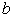is practically constant. The calibrated coefficient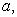on the other hand, varies with latitude. Driedger 4 in 1970 showed that Ångström-Prescott coefficients evolve in a polynomial function of degree two depending on the day of the year. Sfeir 5 in 1981 also showed that the Ångström-Prescott coefficient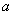is a sinusoidal function of the day of the year. Neuwirth 6 in 1980 came to the conclusion that the Ångström-Prescott coefficients are polynomial functions of order two of the altitude of the stations. Unfortunately the work of Crivelli 7 in 1973 had already contradicted Neuwirth's results. Glover and McCulloch 8 in 1958 suggested a linear relationship between the sum of the Ångström-Prescott coefficients and the optical air mass. Frère et al. 9 in 1975 showed that Ångström-Prescott coefficients are correlated with the mean relative sunshine duration by a quadratic function. Inspired by this idea, Rietveld 10 in 1978 established a linear relationship between Ångström-Prescott coefficients and the mean relative sunshine duration. Stigter and Waryoba 11 in 1981 tested Rietveld's formula in East Africa and found good results. In the same way, researchers like Da Mota et al. 12 in 1977, Flocas 13 in 1980, Malek 14 in 1979 had already used these relations or tested the relations proposed by Rietveld respectively in Brazil, Greece and Iran and obtained interesting results. Obviously the linear correlation coefficient of this relation is better when the stations used are not too dispersed. Gariepy 15 in 1980 proposed an empirical relationship linking mean air temperature and precipitation. In 1984, Kilic and Ozturk 16 showed that Angstrom-Prescott coefficients are closely related to altitude, latitude and declination. Zabara 17 for his part found, a polynomial function of order three of the relative sunshine duration in 1986. Gopanathan 18 in 1988 proposed a relation linking the latitude, the altitude and the average relative sunshine duration. Zhou et al., in 2005 19 studied the dependence of Angstrom-Prescott coefficients on latitude and altitude in China. In 2009, Liu et al. 20 proposed two relations in their study: one giving the coefficientas a quadratic function of the mean temperature as well as the sum of the two coefficients; the other relates the two coefficients to altitude. According to the authors, the second relation gives good results for altitudes located around 2125 m.

The choice of a model is based on the availability of data related to the parameters that compose it. In Côte d'Ivoire, sunshine duration and solar radiation data are only available from a few agro-meteorological stations. To extend the estimation of Ångström-Prescott coefficients to any site in the country, it comes down to find a simple relationship linking them to the parameters of each site. To do so, we opted for the idea of Zhou et al., 19. In fact, in a first study, we have already determined the Ångström-Prescott coefficients in nine (9) meteorological stations which will serve as a basis to construct a general formulation of them for each site in Côte d’Ivoire.

### 2. Methods and Material

2.1. Theoretical Aspect

The Ångström-Prescott equation establishes a linear relationship between the sky clearness index and the relative sunshine duration for a site and for a given period. To determine the Ångström-Prescott coefficients involved in this equation, it is necessary to have sunshine durations and solar radiation data corresponding to this period. However, in Côte d’Ivoire, as in certain regions of the world, these measures are not all time available. To overcome this lack of data, correlations are established between the Ångström-Prescott coefficients and the relevant parameters that influence them depending on the site. Table 1 below gives the general equations of the Ångström-Prescott coefficients obtained by a review of the literature. Liu et al. 2 have in their work studied the performance of these models in China. Readers are invited to refer to their study for more details. For our work and depending on the available data, we opted for the model of Zhou et al. 19 which links each Ångström-Prescott coefficient to the latitude and the altitude of station as follows: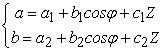(1)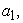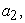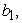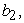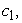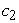are constant coefficients to be calibrated,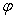denotes the latitude and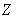the altitude.

To carry out the study, we used data from nine (9) radiometric stations in Côte d'Ivoire covering 2006 to 2010. These data including the sunshine duration and the monthly average daily global solar radiation on horizontal surface collected by Société d’Exploitation et de Développement Aéroportuaire, Aéronautique et Météorologique (SODEXAM) in charge of measuring meteorological parameters in Côte d’Ivoire are used at this purpose. Figure 1 shows the geographic positions of the selected meteorological stations.

• Figure 1. Geographical positions of selected weather stations (SODEXAM)

Côte d’Ivoire is a West Africa country located between latitudes 4°N and 10°N. It is situated in two main climatic zones: the humid tropical climate in the south and the Sudanese climate in the north. Overall, Côte d'Ivoire has a relatively flat and slightly uneven relief, made up of plains in the North and plateaus in the South. Only the region of Tonkpi (Man) has mountainous relief. The mountain peaks do not exceed 1,300 meters in altitude, with the exception of Mount Nimba, located on the border with Guinea which culminates at 1,752 meters.

Table 2 shows the geographic coordinates of the sites as well as the measurement periods.

The coefficients of the Ångström-Prescott equation,andwere determined by linear regression using the least squares method relating the monthly mean daily clearness index to the relative sunshine duration at each station. Theandvalues are obtained from data covering the period 2006 to 2010, collected annually in each weather station. The results obtained in a recent study are shown in Table 3.

From the calibration results obtained, we parameterized the Ångström-Prescott coefficients (1) in order to extend their determination to each site in the country even where no measurement is available. Multiple regressions are used to determine the parameters of the equations contained in (1) and the following results are obtained: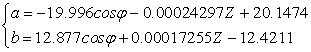(2)

In these equations, the altitude z is expressed in meters (m).

2.3. Analysis Methods

After determining the parameters of the Ångström-Prescott coefficients, the model is evaluated by comparing the calibrated and estimated values. The performance of the model is determined through the mean square error (RMSE), the mean bias error (MBE), the mean absolute bias error (MABE), the statistical t-test (t-stat) and the Nash-Sutcliffe coefficient (NSE) defined as follows: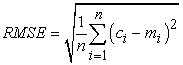(3)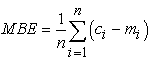(4)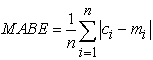(5)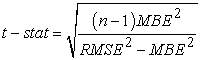(6)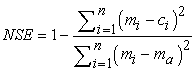(7)

where,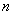is the number of data used,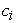the estimated values,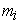the calibrated values and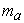is the mean of calibrated values.

RMSE and MBE are the most common indicators used to compare models in the field of solar radiation estimation. The RMSE provides information on the short-term performance of the correlations by allowing a term-by-term comparison of the actual deviation between the estimated and calibrated values. The MBE on the other hand, shows the overestimation or the underestimation of the calculated values. Low values of RMSE and MBE are desired but depending on the data, some fluctuations may be observed. Togrul 21 showed that the isolated use of RMSE and MBE is not an adequate method for evaluating the performance of the model and concluded that the addition of the statistical t-test gave reliable and explanatory results for the evaluation and comparison of solar radiation models 22. Jacovides 23, 24 also demonstrated that the separate use of the RMSE and MBE indicators can lead to poor selection of the best model. He generally recommended using the statistical t-test in conjunction with RMSE and MBE to reliably assess model performance. The MABE measures the average of the absolute differences between predicted and calibrated values. Low values of MABE are also desired. The statistical t-test is determined from the mean squared error and the mean bias error. In this article, as suggested, we use the statistical t-test in conjunction with the RMSE and the MBE, for the evaluation of the model, assuming that the smaller the value of the statistical t-test, the better the performance of the model. To determine if a model is statistically significant, the absolute value of the calculated statistical t-test must be less than the critical value of the t-test, obtained from the tables. Referring to the number of data used (n = 9) and at a 95% significance level, the critical value of the statistical t-test is 1.860 for a one-sided test. In regression procedures, NSE is equivalent to the coefficient of determination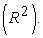Values of the NSE nearer to 1, suggest a model with more predictive skill.

### 3. Results and Discussion

The results of the Ångström-Prescott coefficients predicted in the nine (9) meteorological stations are presented in Table 4. The various indicators and statistical tests are also given in Table 5. According to Table 4, the values of the estimated coefficientrange from 0.2056 to 0.2636 with coefficient of variability of 2.9 % while those ofrange from 0.3851 to 0.4184 with coefficient of variability of 8.5 %. Obviously, the predicted Ångström-Prescott coefficients cover practically the same intervals as those calibrated. By observing Table 5, the values of NSE close to 1 (0.7653197 and 0.99734601) of the two coefficients, indicate that the estimated values are close to the calibrated ones. This remark is also confirmed by the comparative bar graphs of the estimated and calibrated coefficients in Figure 2 and Figure 3 and the RMSE in Table 5 indicates that the absolute mean deviation between the estimated and calibrated values is 0.0063 forand 0.0094 for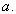Those values are satisfactory for they have no large magnitude. The MBE in Table 5 shows that the model slightly underestimates the values of the Ångström-Prescott coefficients.

• Figure 2. Comparative bar graphs of the estimated and calibrated coefficients b
• Figure 3. Comparative bar graphs of estimated and calibrated a coefficients

Meanwhile Figure 2 and Figure 3 show that the model predicts coefficientbetter than coefficientIn fact, the statistical indicators of coefficientare in absolute value much lower than those of coefficientThis confirms that the estimate of the coefficientis better than that of the coefficientThe Ångström-Prescott coefficients should be used to estimate the monthly mean daily global irradiation in different sites of Côte d’Ivoire. The accuracy of their values leads to the accuracy of the estimated solar radiation. As underlined by Liu et al. 2 the accurate prediction of coefficientis more important than that of coefficientin estimating global solar radiation. Therefore, priority should be given to parameter models having higher accuracy for coefficientTo improve the accuracy of the coefficientwe have correlated it to the coefficientby a polynomial function of degree two as shown in Figure 4.

• Figure 4. Change in coefficient a as a function of coefficient b

The relation obtained is: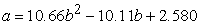(8)

With this new equation, the statistical indicators calculated are shown in Table 6 and the comparative bar graphs of the change in coefficient a is given in Figure 5.

• Figure 5. Comparative bar graphs of the estimated and calibrated a coefficients

Figure 5 shows a clear improvement in the estimate of the coefficientby relation (8) compared to Figure 3. The statistical indicators in Table 6 show a significant drop in MABE and RMSE of 58.02 % and 46.70 % respectively. Thus, the estimated values of the coefficientare close to those calibrated. The positive value of the MBE indicates that the model overestimates the values of the coefficientbut this overestimation is slight in magnitude.

Ultimately, this second method improves the accuracy of coefficientThis analysis allows us to retain the following two equations: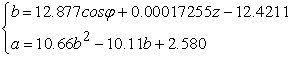(9)

In that use, a two-step procedure is needed: predicting coefficientfirst and then coefficientin the second step by substituting the value ofin the expression ofThese equations validly estimate the Angström-Prescott coefficients over the entire extent of Côte d’Ivoire by knowing for each site its latitude and altitude.

### 4. Conclusion

Attempts have been made in this work to propose parametrized annual Angström-Prescott coefficients in Côte d'Ivoire using data from nine (9) weather stations, sampled in monthly mean daily global radiation and sunshine duration. The Angström-Prescott coefficients are correlated to latitude and altitude. The performance of the models was evaluated using some errors indicators. The study found that the accuracy of coefficientis less that this of coefficient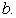To improve the accuracy of coefficienta polynomial approach is suggested linking it to coefficientThe global results obtained show a strong correlation with a high determination coefficient for each parameter confirmed by values of NSE close to 1. These results indicate that the two equations proposed in the model can be used satisfactorily for the estimation of Angström-Prescott coefficients in any site in Côte d'Ivoire.

### Nomenclature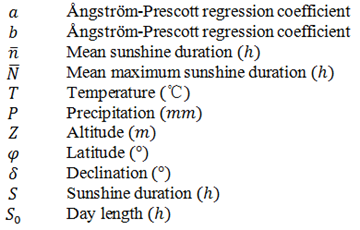### References

Published with license by Science and Education Publishing, Copyright © 2021 Maurice Aka Djoman, Ferdinand Wanignon Fassinou and Augustin MemelèdjeThis work is licensed under a Creative Commons Attribution 4.0 International License. To view a copy of this license, visit http://creativecommons.org/licenses/by/4.0/